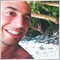# Find the biggest, 2nd biggest and 3rd biggest orders in Profit in Orderstotal()11

Hi,

Can anybody help me please ?

I know how to find the biggest order in Profit using a for loop.

But i dont know how to find the 2nd and 3rd biggest orders in profit.

Amir4163

Find the biggest order and save its ticket to a variable.

Find again the biggest order with ticket != from that variable and save its ticket to a new variable.

Find again the biggest order with ticket != from that 2 variables.

You found the 3rd biggest order.2358

Show us how u find the biggest order then we will work from there11

```double BiggestProfit()
{
double Profit = 0;

for(int i = 0; i <= OrdersTotal(); i = i + 1)
{
{
if(OrderProfit() > Profit)
{
Profit         =  OrderProfit();
BiggestTicket  =  OrderTicket();
}
}
}
return(Profit);
}
```

Hi Guys,

This gives me the biggest profit.

Amir2358

Now after the first run we have biggest profit and that order number is i. So we save i as biggest.

So we run it again and skip this time with line

```if(i == biggest)
continue; ```

Then we get second

Re run loop with line

```if(i== second || i == biggest)
continue;```

Done. So we have loop

```int biggest, second, third;
//Equal them to 0

do
Your_Function(&biggest, &second,&third)
while (third==0)

//This is just a logic idea and is not order to syntax wise I hope u see the solution. Though there better and faster ways```